NCERT Solutions for Class 6 Maths Chapter 3 Playing with Numbers Ex 3.3

# NCERT Solutions for Class 6 Maths Chapter 3 Playing with Numbers Ex 3.3

## NCERT Solutions for Class 6 Maths Chapter 3 Playing with Numbers Ex 3.3

NCERT Solutions for Class 6 Maths Chapter 3 Playing with Numbers Ex 3.3 are the part of NCERT Solutions for Class 6 Maths. Here you can find the NCERT Solutions for Class 6 Maths Chapter 3 Playing with Numbers Ex 3.3.

### Ex 3.3 Class 6 Maths Question 1.

Using divisibility tests, determine which of the following numbers are divisible by 2, by 3, by 4, by 5, by 6, by 8, by 9, by 10, by 11 (Say, Yes or No):

Solution:

### Ex 3.3 Class 6 Maths Question 2.

Using divisibility tests, determine which of following numbers are divisible by 4; by 8:
(a) 572
(b) 726352
(c) 5500
(d) 6000
(e) 12159
(f) 14560
(g) 21084
(j) 2150
(h) 31795072
(i) 1700

Solution:
(a) Given number = 572
(i) Divisibility by 4
Here, the number formed by the last two digits of the given number is 72.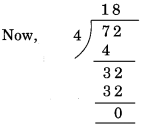Remainder = 0. Hence, 572 is divisible by 4.

(ii) Divisibility by 8
Here, the number formed by the last three digits of the given number is 572.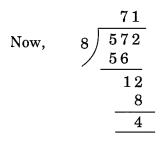Remainder = 4. Hence, 572 is not divisible by 8.

(b) Given number = 726352
(i) Divisibility by 4
Here, the number formed by the last two digits of the given number = 52.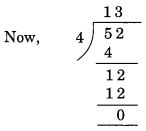Remainder = 0.
Hence, 726352 is divisible by 4.

(ii) Divisibility by 8
Here, the number formed by the last three digits of the given number = 352Remainder = 0.
Hence, 726352 is divisible by 8.

(c) Given number = 5500
(i) Divisibility by 4
Here, the last two digits of the given number are 00. Hence, 5500 is divisible by 4.
(ii) Divisibility by 8
Here, the number formed by the last three digits of the given number = 500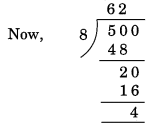Remainder = 4. Hence, 5500 is not divisible by 8.

(d) Given number = 6000
(i) Divisibility by 4
Here, the last two digits of the given number are 00.
Hence, 6000 is divisible by 4.

(ii) Divisibility by 8
Here, the last three digits of the given number are 000.
Hence, 6000 is divisible by 8.

(e) Given number = 12159
(i) Divisibility by 4
Here, the number formed by last two digits of the given number = 59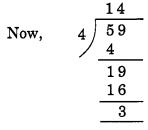Remainder = 3.
Hence, 12159 is not divisible by 4.

(ii) Divisibility by 8
Here, the number formed by the last three digits of the given number = 159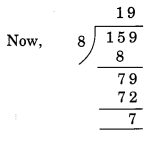Remainder = 7.
Hence, 12159 is not divisible by 8.

(f) Given number = 14560
(i) Divisibility by 4
Here, the number formed by the last two digits of the given number = 60.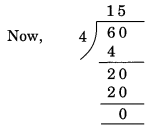Remainder = 0.
Hence, 14560 is divisible by 4.

(ii) Divisibility by 8
Here, the number formed by the last three digits of the given number = 560.
560 ÷ 8 = 70
Remainder = 0.
Hence, 14560 is divisible by 8.

(g) Given number = 21084
(i) Divisibility by 4
Here, the number formed by the last two digits of the given number = 84.Remainder = 0. Hence, 21084 is divisible by 4.

(ii) Divisibility by 8
Here, the number formed by the last three digits of the given number = 084.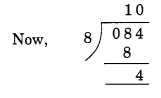Remainder = 4.
Hence, 21084 is not divisible by 8.

(h) Given number = 31795072
(i) Divisibility by 4
Here, the number formed by the last two digits of the given number = 72.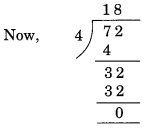Remainder = 0. Hence, 31795072 is divisible by 4.

(ii) Divisibility by 8
Here, the number formed by the last three digits of the given number = 072.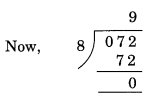Remainder = 0. Hence, 31795072 is divisible by 8.

(i) Given number = 1700
(i) Divisibility by 4
Here, the last two digits of the given number are 00. Hence, 1700 is divisible by 4.
(ii) Divisibility by 8
Here, the number formed by the last three digits of the given number = 700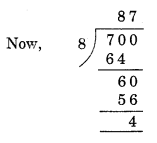Remainder = 4. Hence, 1700 is not divisible by 8.

(j) Given number = 2150
(i) Divisibility by 4
Here, the number formed by the last two digits of the given number = 50.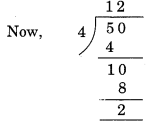Remainder = 2. Hence, 2150 is not divisible by 4.

(ii) Divisibility by 8
Here, the number formed by the last three digits of the given number = 150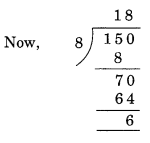Remainder = 6. Hence, 2150 is not divisible by 8.

### Ex 3.3 Class 6 Maths Question 3.

Using divisibility tests, determine which of the following numbers are divisible by 6:
(a) 297144
(b) 1258
(c) 4335
(d) 61233
(e) 901352
(f) 438750
(g) 1790184
(h) 12583
(i) 639210
(j) 17852

Solution:
We know that a number is divisible by 6, if it is divisible by 2 and 3 both.
(a) Given number = 297144
The given number 297144 has even digit, that is, 4 at its one’s place.
So, it is divisible by 2.
The sum of all the digits of 297144 = 2 + 9 + 7 + 1 + 4 + 4 = 27
which is divisible by 3.
Hence, the given number is divisible by 6.

(b) Given number = 1258
The given number 1258 has even digit, i.e., 8 at its one’s place.
So, it is divisible by 2.
The sum of all digits of 1258 = 1 + 2 + 5 + 8 = 16 which is not divisible by 3.
Hence, the given number is not divisible by 6.

(c) Given number = 4335
The digit at one’s place of the given number is not even.
So, it is not divisible by 2.
The sum of all the digits of 4335 = 4 + 3 + 3 + 5 = 15 which is divisible by 3.
Since the given number is not divisible by both 2 and 3, therefore, it is not divisible by 6.

(d) Given number = 61233
The digit at one’s place of the given number is not even.
So, it is not divisible by 2.
The sum of the digits of the given number 61233 = 6 + 1 + 2 + 3 + 3 = 15 which is divisible by 3.
Since the given number is not divisible by both 2 and 3, therefore, it is not divisible by 6.

(e) Given number = 901352
The digit at one’s place of the given number is even.
So, it is divisible by 2.
The sum of all the digits of the given number 901352 = 9 + 0 + 1 + 3 + 5 + 2 = 20 which is not divisible by 3.
Since the given number is not divisible by both 2 and 3, therefore, it is not divisible by 6.

(f) Given number = 438750
The digit at one’s place of the given number is 0. So, it is divisible by 2.
The sum of all the digits of the given number 438750
= 4 + 3 + 8 + 7 + 5 + 0 = 27 which is divisible by 3.
Hence, the given number is divisible by 6.

(g) Given number = 1790184
The digit at one’s place of the given number is even, i.e., 4.
So, it is divisible by 2.
The sum of all the digits of the given number 1790184
= 1 + 7 + 9 + 0 + 1 + 8 + 4 = 30 which is divisible by 3.
Hence, the given number is divisible by 6.

(h) Given number = 12583
The digit at one’s place of the given number is odd, i.e., 3.
So, it is not divisible by 2.
The sum of all the digits of the given number 12583
= 1 + 2 + 5 + 8 + 3 = 19 which is not divisible by 3.
Hence, the given number is not divisible by 6.

(i) Given number = 639210
The digit at one’s place of the given number is 0.
So, it is divisible by 2.
The sum of all the digits of the given number 639210
= 6 + 3 + 9 + 2 + 1 + 0 = 21 which is divisible by 3.
Hence, the given number is divisible by 6.

(j) Given number = 17852
The digit at one’s place of the given number is even, i.e., 2.
So, it is divisible by 2.
The sum of all the digits of the given number 17852
= 1 + 7 + 8 + 5 + 2 = 23 which is not divisible by 3.
Hence, the given number is not divisible by 6.

### Ex 3.3 Class 6 Maths Question 4.

Using divisibility tests, determine which of the following numbers are divisible by 11:
(a) 5445
(b) 10824
(c) 7138965
(d) 70169308
(e) 10000001.

Solution:

We know that a number is divisible by 11, if the difference between the sum of the digits at odd places (from the right) and the sum of the digits at even places (from the right) of the number is either 0 or divisible by 11.
(a) Given number = 5445
The sum of the digits at odd places = 5 + 4 = 9
The sum of the digits at even places = 4 + 5 = 9
Difference = 9 – 9 = 0
Hence, the given number is divisible by 11.

(b) Given number = 10824
The sum of the digits at odd places = 4 + 8 + 1 = 13
The sum of the digits at even places = 2 + 0 = 2
Difference = 13 – 2 = 11 which is divisible by 11.
Hence, the given number is divisible by 11.

(c) Given number = 7138965
The sum of the digits at odd places = 5 + 9 + 3 + 7 = 24
The sum of the digits at even places = 6 + 8 + 1 = 15
Difference = 24 – 15 = 9 which is not divisible by 11.
Hence, the given number is not divisible by 11.

(d) Given number = 70169308
The sum of all the digits at odd places = 8 + 3 + 6 + 0 = 17
The sum of all the digits at even places = 0 + 9 + 1 + 7 = 17
Difference = 17 – 17 = 0
Hence, the given number is divisible by 11.

(e) Given number = 10000001
The sum of all the digits at odd places = 1 + 0 + 0 + 0 = 1
The sum of all the digits at even places = 0 + 0 + 0 + 1 = 1
Difference = 1 – 1 = 0
Hence, the given number is divisible by 11.

### Ex 3.3 Class 6 Maths Question 5.

Write the smallest digit and the greatest digit in the blank space of each of the following numbers so that the number formed is divisible by 3.
(a) ___6724
(b) 4765___2

Solution:
We know that a number is divisible by 3, if the sum of all the digits of the number is also divisible by 3.
(a) ___6724
The sum of the digits = 4 + 2 + 7 + 6 = 19
The smallest digit to be placed is blank space = 2
Then the sum = 19 + 2 = 21 which is divisible by 3.
The greatest digit to be placed in blank space = 8
Then the sum = 19 + 8 = 27 which is divisible by 3
Hence, the required digits are 2 and 8.

(b) 4765___2
The sum of digits = 2 + 5 + 6 + 7 + 4 = 24
The smallest digits to be place in blank space = 0
Then the sum = 24 + 0 = 24 which is divisible by 3.
The greatest digit to be placed in blank space = 9.
Then the sum = 24 + 9 = 33 which is divisible by 3.
Hence, the required digits are 0 and 9.

### Ex 3.3 Class 6 Maths Question 6.

Write a digit in the blank space of each of the following numbers so that the numbers formed is divisible by 11.
(a) 92___389
(b) 8___9484

Solution:
(a) 92___389
The sum of the digits at odd places = 9 + 3 + 2 = 14
The sum of the digits at even places = 8 + ( ) + 9 = 17 + ( )
Difference = 17 + ( ) – 14 = ( ) + 3
For the given number to be divisible by 11,
( ) + 3 = 11
( ) = 11 – 3 = 8
So, the missing digit = 8
Hence, the required number is 928389.

(b) 8___9484
The sum of the digits at odd places = 4 + 4 + ( ) = 8 + ( )
The sum of the digits at even places = 8 + 9 + 8 = 25
Difference = 25 – [8 + ( )]
= 25 – 8 – ( ) = 17 – ( )
For the given number to be divisible by 11,
17 – ( ) = 11
17 – 11 = ( ) or ( ) = 6
So, the missing digit = 6
Hence, the required number is 869484.

You can also like these:

NCERT Solutions for Maths Class 7

NCERT Solutions for Maths Class 8

NCERT Solutions for Maths Class 9

NCERT Solutions for Maths Class 10

NCERT Solutions for Maths Class 11

NCERT Solutions for Maths Class 12